﻿ A New Graphical User Interface for Teaching NMR

### A New Graphical User Interface for Teaching NMROPEN ACCESSPEER-REVIEWED

## A New Graphical User Interface for Teaching NMR

Jamal Hassan1,, Ahmad Al Nabulsi2

1Department of Applied Mathematics and Sciences, Khalifa University, UAE

2Department of Electronic Engineering, Khalifa University, UAE

### Abstract

The classical Nuclear Magnetic Resonance (NMR) vector model is known to be very effective and useful in teaching basic NMR 1D pulse sequences such as spin echo. For more complicated spin systems, density matrix calculation is often used. This method is known to be very complicated and not easy to follow after couple steps. Students usually get lost within the calculation and loose the physical meaning of the effect of different NMR pulses and other operations (chemical shifts, scalar-couplings) on the spin-system under investigation. As a compromise, another methodology has been developed which depends on the spin angular momentum operators. This is known as product operator formalism which involves less mathematical calculations, but still requires the user to know the correct outcome of different NMR pulses, scalar couplings and chemical shifts on different spin operators. We have developed product operator graphical user interface (OP-GUI) using MATLAB software that is capable of calculating product operators of up to four-coupled ½ spin systems. The effect of NMR pulses, scalar-coupling and chemical shifts on the spin system under investigation can be easily calculated. In this paper, several examples are given as prove of concept to show the capability and flexibility of the obtained OP-GUI in dealing with spin systems. We believe the obtained calculator is an effective teaching tool for new students in NMR and will help them to better understand and interpret complicated NMR pulse sequences applied on up to four weakly coupled ½ spin systems.

### At a glance: Figures

12
Prev Next

• Hassan, Jamal, and Ahmad Al Nabulsi. "A New Graphical User Interface for Teaching NMR." American Journal of Educational Research 2.5 (2014): 240-244.
• Hassan, J. , & Nabulsi, A. A. (2014). A New Graphical User Interface for Teaching NMR. American Journal of Educational Research, 2(5), 240-244.
• Hassan, Jamal, and Ahmad Al Nabulsi. "A New Graphical User Interface for Teaching NMR." American Journal of Educational Research 2, no. 5 (2014): 240-244.

 Import into BibTeX Import into EndNote Import into RefMan Import into RefWorks

### 1. Introduction

In the past couple decades a large number of pulse NMR experiments have been developed to extract more information from the spin system under investigation. For the design, analysis and teaching these pulses, different methods have been followed. Many of the simple NMR pulse sequences were successfully explained using classical or semi-classical vector model  which relies on hand-waving to study the effect of different pulses on the magnetization vectors on the x, y, z directions. Unfortunately, this method has limitation on complicated pulse sequences. Therefore, for the analysis of more complex pulse experiments density matrix approach was often used [2, 3, 4, 5]. This method involves extensive matrix manipulation and calculation. So often, one gets lost after couple introductory steps and gets away from the physical intuition. As a midway between these two approaches the product operator formalism has been introduced. Product operators rely on using angular momentum operators instead of matrices and originally intended to simplify the calculation. This technique brought a better tool for understanding many now used NMR pulse sequences that cannot be analyzed using the normal vector model. Product operator is a simple quantum mechanical method has been developed for the analytical description of multi-pulse NMR experiments on the weakly coupled spin systems [6-14]. There are many examples that appeared in the literature using product operators but mostly for simple two-spin systems and to less extend to three-spin systems. According to the authors of this study, there are still no examples of higher order spin groups such as four- spin systems treated in the literature using this approach.

In this article, we present an easy alternative tool for teaching various NMR pulse sequences to undergraduate students. This tool is Operator Product Graphical User Interface OP-GUI developed using MATLAB software that is capable to deal with up to four-weakly ½ spin systems. The OP-GUI can be used effectively in teaching NMR students the effect of different pulse sequences on a spin system under investigation. The user can start from any of the known initial product operators to see the effect of the specified or arbitrary NMR pulses, chemical shift and coupling between the spin groups. The final result can be simplified to give better interpretation for the expected NMR signal.

### 2. Background

For a single ½ spin there are four spin operators; two transverse magnetization spin operators Ix and Iy, the equilibrium magnetization spin operators Iz and the unity operator E/2 (where ½ is used for normalization purposes). It is not difficult to follow the effect of an NMR pulse on these operators using vector model.

For example a pulse of θ degrees along x-axis will tip the equilibrium magnetization (Mz in vector model) by θ degrees. Given as: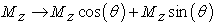However, in product operator, spin operators Ix, Iy and Iz are used instead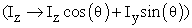. The result shows the initial operator (Iz, in this case) is modified with cos(θ) term plus a new operator (Iy, in this example) modified by the sin(θ) term. In fact, this is the case in most of the transformation in product operators. In addition, for a single spin, chemical shift evolution in the transverse plane, on Iy for instance, can be written as: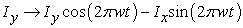. Where t is the time interval in which the chemical shift is active and the whole term 2πwt represents the angle of rotation.

For two spins (I1 and I2) there are 16 possible product operators; I1z, I2z are unobservable z-components of magnetization. I1x, I1y, I2x, I2y are observable x and y components of the magnetization. 2I1z I2z is the unobservable longitudinal two-spin order. 2I1x I2z, 2I1y I2z, 2I1z I2x, 2I1z I2y are the observable antiphase magnetization. 2I1x I2x, 2I1x I2y, 2I1y I2x, 2I1y I2y are non-observables two-spin coherences. For two-spin system (and higher orders), the effect of an NMR pulse depends on which spin the pulse is applied. For example, if a pulse of θ degrees is applied on I1 along x-axis will leave I2 unaffected as shown in the following example:

An applied pulse of θ degrees on I1 (of 2I1z I2z) along x-axis written as: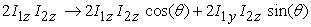Coupling between the two spins is another process that might occur. Here the coupling evolution is given as: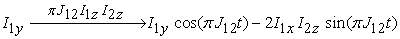where J12 is the coupling constant and t is the evolution time for the coupling between the two spins (I1 and I2). For three or four weakly coupled ½ spins, transformations under different pulses, chemical shifts and couplings are similar to the previous cases. For example in the three-spin system, the effect of coupling between spin 1 and 3 on the 2I1x I2z leave the I2z unchanged.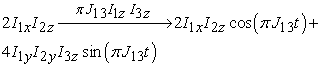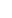In general, the following rules are applied: i) only product operators with one transverse operator (Ix or Iy) are observable, ii) product operators with one transverse operator (Ix or Iy) and any number of longitudinal operator Iz evolve into observable operator as long as the transverse spin operator is scalar-coupled to all the longitudinal ones and iii) all other product operators are not observable, and do not evolve into observable operators.

### 3. The Obtained OP-GUI

The obtained OP-GUI is written and developed using MATLAB GUI development environment (GUIDE), based on a set of M-files each representing one of the program functions. Those M-files uses the power of MATLAB functions and symbolic toolbox in computing NMR product operator formalism. The detail of the spin operator transformation is given previously in many references [6, 12]. The OP-GUI has different sections as shown in Figure 1. The top part is the input section where the initial spin operators need to be typed in. The notation used for different spins are I1, I2, I3 and I4 while spin operator components are typed in as I1x, I1y and I1z and no subscriptions are used. The “Applied NMR Pulse” section shows different pulses that can be applied to up to 4-spins with specified or arbitrary angles θ. The value of θ in degrees can be entered in the “Substitute Theta” area for further calculation. The “J-Coupling” section gives the user the flexibility in applying the scalar coupling on four-spin groups independently. This is very useful because in many complex systems the scalar coupling among different spin groups might have different strengths and/or with different coupling durations. The drop-menu of the “J-Coupling” section includes different coupling durations (1/J, 1/2J, 1/4J and 1/8J) that can be chosen by the user for the final calculation. Information about chemical shift on any spin group up to four (chosen from the drop-menu) can be obtained by clicking on the “Chemical Shift” button with the option to simplify the result if the time interval of the chemical shift is known. In addition to that, the GUI provides the option to eliminate any unobservable product operator that might appear in the final answer by using the “Spin Operator Elimination” section. By clicking on any of the nine product operators in this section the term (of the final answer) includes the chosen operator will be eliminated. This simplifies the final result and provides the user more information on the predicted NMR signal. The output of the OP-GUI appears on the “Result” section which can be copied by using the normal copy-paste method for further investigation. It is important to mention that, the user can start from any known product operators to see the effect of different operations from NMR pulses, chemical shifts, or scalar coupling to get better interpretation of the spin operator transformation.

### 4. Using the PO-GUI in Teaching NMR Experiments

An easy example is shown in the “Result” section of Figure 1 of a scalar coupling between spins 1 and 3 (in a three-spin system). This is applied on the initial operator 2I1x I2z without affecting I2z as expected from the spin operator formalism and density matrix calculation which both require a lot of calculation. This example shows that, the user can start from any product operator to apply pulses with different durations and directions, scalar couplings, and chemical shifts. This gives students an excellent way to understand the effect of such processes on particular product operator and give them opportunity to modify or design a particular NMR pulse sequence to obtain a required NMR signal output. Below we will show several complete examples using the obtained PO-GUI. The first example is the spin-echo experiment which of course can be taught using the typical vector model. Later on, we will show other examples that cannot be interpreted using the classical vector model but successfully explained by our PO-GUI.

Figure 1. The obtained operator product graphical user interface (OP-GUI)
4.1. Spin-echo Experiment

The product operator formalism can be used for prediction of the NMR signal in the spin-echo experiment Here we will use our PO-GUI to obtain the expected outcome. Figure 2 shows the pulse sequence for this experiment which includes two pulses; one of 90ox and the other of 180ox separated by an interval of time.

The acquisition of the NMR signal starts after the second pulse. The user starts to use the OP-OGI by simply entering the initial spin operator (I1z) in the input section of and then apply the pulse sequence (according to Figure 2) till gets to the final result. The solution steps are copied directly from the output section of the OP-GUI and are pasted below:

•  ► I1z

•  Apply pulse 90_1x

•  ► -I1y

•  Apply chemical shift

•  ► I1y*cos(2*π*t*ω) - I1x*sin(2*π*t*ω)

•  Apply J_12 coupling

•  ► I1y*cos(2*π*J_12*t)*cos(2*π*t*ω) - I1x*sin(2*π*t*ω)*cos(2*π*J_12*t) - 2*I1x*I2z*sin(2*π*J_12*t)*cos(2*π*t*ω) - 2*I1y*I2z*sin(2*π*t*ω)*sin(2*π*J_12*t)

•  Apply pulse 180_1x

•  ► 2*I1y*I2z*sin(2*π*t*ω)*sin(2*π*J_12*t) - I1x*sin(2*π*t*ω)*cos(2*π*J_12*t) - 2*I1x*I2z*sin(2*π*J_12*t)*cos(2*π*t*ω) - I1y*cos(2*π*J_12*t)*cos(2*π*t*ω)

•  Apply J_12 coupling

•  ► - I1y*cos(2*π*J_12*t)^2*cos(2*π*t*ω) - I1x*sin(2*π*t*ω)*cos(2*π*J_12*t)^2 - I1y*sin(2*π*J_12*t)^2*cos(2*π*t*ω) - I1x*sin(2*π*t*ω)*sin(2*π*J_12*t)^2

•  Apply J_coupling time t=1/2J

•  ► - I1y*cos(2*π*t*ω) - I1x*sin(2*π*t*ω)

•  Apply t substitution

•  ► -I1y

It is obvious that the predicted NMR signal will be on the –y axis as expected if one uses the typical vector model method .

4.2. DEPT Experiment for Two-Spin System

In this section a more advanced NMR experiments will be demonstrated using our calculator. Obviously these cannot be explained by using the typical NMR vector model and require extensive calculation if one uses either density matrix or operator product formalism. Distortionless Enhancement by Polarization Transfer (DEPT) experiment is widely used to simplify spectra of carbonyl groups in complex molecules [6, 15, 16, 17]. DEPT experiment results in an enhancement of the intensity of the low natural abundance X-nuclei (such as carbon) by using nuclei of high natural abundance (such as hydrogen). Both nuclei are irradiated with specific pulses but the acquisition is on the X-nuclei channel as shown in Figure 3.

The intensity of the X-nuclei signal depends on the length of the last Hydrogen pulse (θ pulse) and on the number of protons coupled to the X-nuclei. Below we will demonstrate the use of our calculator for different number of spin groups starting with two-spin groups (CH) as shown below. In the following I1 refer to Hydrogen and I2 refer to Carbon nuclei.

•  Initial Spin Operator

•  ► I1z

•  Apply pulse 90_1y

•  ► I1x

•  Apply J_12 coupling

•  ► I1x*cos(2*π*J_12*t) + 2*I1y*I2z*sin(2*π*J_12*t)

•  Apply J_coupling time t=1/2J

•  ► 2*I1y*I2z

•  Apply pulse 90_2y

•  ► 2*I2x*I1y

•  Apply J_12 coupling

•  ► 2*I2x*I1y

•  Apply J_coupling time t=1/2J

•  ► 2*I2x*I1y

•  Apply pulse θ_1x

•  ► 2*I2x*(I1y*cos(θ) + I1z*sin(θ))

•  Apply J_12 coupling

•  ► I2y*sin(2*π*J_12*t)*sin(θ) + 2*I2x*I1y*cos(θ) + 2*I2x*I1z*cos(2*π*J_12*t)*sin(θ)

•  Apply J_coupling time t=1/2J

•  ► I2y*sin(θ) + 2*I2x*I1y*cos(θ)

•  Eliminate the non-observable operator I_2x

•  I2y*sin(θ)

The final NMR signal is modulated by sin(θ) as expected from the product operator formalism or density matrix calculation [14, 18].

4.3. DEPT Experiment for Three-spin System

For three-spin group, I1 and I2 represent the two hydrogen spin groups (in CH2) and I3 represents carbon (in CH2) and the scalar coupling is between I1, I3 and I2, I3. The initial spin operator is the two hydrogen groups (I1z+I2z). To implement the pulse sequence of Figure 3, one needs to apply the top part of the pulses for both I1 and I2. The full solution, using the OP-GUI, is shown below:

•  Initial Spin Operator

•  ► I1z+I2z

•  Apply pulse 90_1y

•  ► I1x + I2z

•  Apply pulse 90_2y

•  ► I1x + I2x

•  Apply J_13 coupling

•  ► I2x + I1x*cos(2*π*J_13*t) + 2*I1y*I3z*sin(2*π*J_13*t)

•  Apply J_23 coupling

•  ► I1x*cos(2*π*J_13*t) + I2x*cos(2*π*J_23*t) + 2*I1y*I3z*sin(2*π*J_13*t) + 2*I2y*I3z*sin(2*π*J_23*t)

•  Apply J_coupling time t=1/2J

•  ► 2*I1y*I3z + 2*I2y*I3z

•  Apply pulse 90_3y

•  ► 2*I3x*I1y + 2*I3x*I2y

•  Apply J_13 coupling

•  ► 2*I3x*I1y + 2*I3x*I2y*cos(2*π*J_13*t) + 4*I2y*I3y*I1z*sin(2*π*J_13*t)

•  Apply J_23 coupling

•  ► 2*I3x*I2y*cos(2*π*J_13*t) + 2*I3x*I1y*cos(2*π*J_23*t) + 4*I2y*I3y*I1z*sin(2*π*J_13*t) + 4*I1y*I3y*I2z*sin(2*π*J_23*t)

•  Apply J_coupling time t=1/2J

•  ► 4*I1y*I3y*I2z + 4*I2y*I3y*I1z

•  Apply pulse θ_1x

•  ► 4*I2y*I3y*(I1z*cos(θ) - I1y*sin(θ)) + 4*I3y*I2z*(I1y*cos(θ) + I1z*sin(θ))

•  Apply pulse θ_2x

•  ► 4*I1y*I3y*cos(θ)*(I2z*cos(θ) - I2y*sin(θ)) + 4*I3y*I1z*cos(θ)*(I2y*cos(θ) + I2z*sin(θ)) - 4*I1y*I3y*sin(θ)*(I2y*cos(θ) + I2z*sin(θ)) + 4*I3y*I1z*sin(θ)*(I2z*cos(θ) - I2y*sin(θ))

•  Apply J_13 coupling

•  ► 2*I3x*I2y*sin(2*π*J_13*t)*sin(θ)^2 - 2*I3x*I2y*cos(θ)^2*sin(2*π*J_13*t) + 4*I1y*I3y*I2z*cos(θ)^2 - 4*I1y*I3y*I2z*sin(θ)^2 + 4*I2y*I3y*I1z*cos(2*π*J_13*t)*cos(θ)^2 - 4*I2y*I3y*I1z*cos(2*π*J_13*t)*sin(θ)^2 - 4*I3x*I2z*cos(θ)*sin(2*π*J_13*t)*sin(θ) - 8*I1y*I2y*I3y*cos(θ)*sin(θ) + 8*I3y*I1z*I2z*cos(2*π*J_13*t)*cos(θ)*sin(θ)

•  Apply J_23 coupling

•  ► 2*I3x*I2y*sin(2*π*J_13*t)*sin(θ)^2 - 2*I3x*I1y*cos(θ)^2*sin(2*π*J_23*t) - 2*I3x*I2y*cos(θ)^2*sin(2*π*J_13*t) + 2*I3x*I1y*sin(2*π*J_23*t)*sin(θ)^2 - 2*I3y*cos(θ)*sin(2*π*J_13*t)*sin(2*π*J_23*t)*sin(θ) + 4*I2y*I3y*I1z*cos(2*π*J_13*t)*cos(θ)^2 + 4*I1y*I3y*I2z*cos(2*π*J_23*t)*cos(θ)^2 - 4*I2y*I3y*I1z*cos(2*π*J_13*t)*sin(θ)^2 - 4*I1y*I3y*I2z*cos(2*π*J_23*t)*sin(θ)^2 - 8*I1y*I2y*I3y*cos(θ)*sin(θ) - 4*I3x*I1z*cos(2*π*J_13*t)*cos(θ)*sin(2*π*J_23*t)*sin(θ) - 4*I3x*I2z*cos(2*π*J_23*t)*cos(θ)*sin(2*π*J_13*t)*sin(θ) + 8*I3y*I1z*I2z*cos(2*π*J_13*t)*cos(2*π*J_23*t)*cos(θ)*sin(θ)

•  Apply J_coupling time t=1/2J

•  ► 2*I3x*I1y*sin(θ)^2 - 2*I3x*I2y*cos(θ)^2 - 2*I3x*I1y*cos(θ)^2 + 2*I3x*I2y*sin(θ)^2 - 2*I3y*cos(θ)*sin(θ) - 8*I1y*I2y*I3y*cos(θ)*sin(θ)

•  Eliminate the non-observable operator I_3x

•  ► - 2.0*I3y*cos(θ)*sin(θ) - 8.0*I1y*I2y*I3y*cos(θ)*sin(θ)

•  Eliminate the non-observable operator I_1y

•  ► -2.0*I3y*cos(θ)*sin(θ)

The final result for the case of three-spin system in DEPT experiment is modulated by cos(θ) sin(θ) . Of course the user can go one step further in using the same pulse sequence four-spin groups (CH3). The final answer in this case is:

•  ► 3.0*I4y*cos(θ)^2*sin(θ)

Which indicates that the result is modulated by (cos(θ))2 sinθ. Both results are predicted by density matrix and product operator formalism . The final answer of the previous examples is graphically represented in Figure 4 which shows how the final NMR signal changes by changing the pulse duration (by changing θ) in the DEPT pulse sequence. For example, for spectra acquired at 45°, all the protonated signals will phase up. In the case of 90° proton pulse, only singly protonated carbon (in CH) will produce. On the other hand, for proton pulse of 135°, CH and CH3 will phase up while CH2 will phase down.

Figure 4. NMR signal intensity in DEPT experiment versus last hydrogen pulse duration, for different spin groups

### 5. Conclusion

The examples shown previously indicate the efficiency and flexibility in using the obtained OP-GUI for up to four weakly coupled ½ spin groups. It is important to mention that the calculator is also applicable for a wide range of other pulse sequences (such as INEPT). It is also obvious how difficult would be for a beginner in NMR to use the product operator formalism or the density matrix method on a piece of paper to get insight into some NMR pulse experiments such as DEPT. We believe the obtained calculator is an effective teaching tool for new students in NMR. It will help them to better understand and to interpret complicated NMR experiments. The tool also provide the user a prediction to what will be the outcome on the observable spin if an existing pulse sequence was modified for a particular NMR application. The latter is very important for designing new pulse sequences for weakly-coupled ½ spins.

### Note

All the source files, executable files and the OP-GUI are available for free, and can be obtained by writing an email to the corresponding author of this article.

### References

  Benn, R., H. Günther , Angew. Chem. 22(5): 350-380. 1983.In article CrossRef  Abragam, A, The Principles of Nuclear Magnetism. London: Oxford University Press, 1963.In article  Slichter, C, Principles of Magnetic Resonance, 2nd Ed. New York: Springer-Verlag, 1990.In article CrossRef  Goldman, M, Quantum Description of High-Resolution NMR in Liquids, Oxford: Clarendon Press, 1990.In article  Ernst, R. R., G. Baudenhausen, A. Wokaun, Principles of Nuclear Magnetic Resonance in One and Two Dimensions, Oxford: Clarendon Press, 1987.In article  Sørensen O.W., G.W. Eich, M.H. Levitt, G. Bodenhausen, R.R. Ernst, Prog. NMR Spectrosc. 16: 163-192, 1983.In article CrossRef  Packer K.J., & K. M. Wright, Molecular Physics. 50(4): 797-813, 1983.In article CrossRef  Howarth M.A., L.Y. Lian, G.E. Hawkes, & K.D. Sales, J. Magn. Reson. 68 (3): 433-452, 1986.In article  Chandrakumar N., S. Subramanian, Modern Techniques in High Resolution FT NMR, New York: Springer, 1987.In article CrossRef  Hore P.J., J.A. Jones, S. Wimperis, NMR: The Toolkit, New York: Oxford Univ. Press, 2000.In article  Van de Ven F.J.M., & C.W. Hilbers, J. Magn. Reson. 54 (3): 512:520, 1983.In article  Shriver J, Concepts Magn. Reson. 4(1): 1-33, 1992.In article CrossRef  Wei G., & R. F. Snider, Magn. Reson. 7(2): 153-163, 1995.In article CrossRef  Sørensen O. W, Prog. NMR Spectrosc. 21:503-569 1989.In article CrossRef  Nolis P., T. Parella, Curr. Analty. Chem. 3(1): 47-68, 2007.In article CrossRef  Doddrell D.M., D.T. Pegg, & M.R. Bendall (1982). J. Magn.Reson. 48 (2): 323-327.In article  Chandrakumar, N, J. Magn. Reson. 60(1): 28-36, 1984.In article  Gheorghe D. Mateescu and Adrian Valerin, 2D NMR Density Matrix and Product Operator Treatment, Prentice Hall, 2003.In article